Reach Us+44-1522-440391
Power of Permutation Tests Using Generalized Additive Models with Bivariate Smoothers | OMICS International
Journal of Biometrics & Biostatistics

Like us on:

All submissions of the EM system will be redirected to Online Manuscript Submission System. Authors are requested to submit articles directly to Online Manuscript Submission System of respective journal.

# Power of Permutation Tests Using Generalized Additive Models with Bivariate Smoothers

Robin Y. Bliss1,2*, Janice Weinberg1, Veronica Vieira3, Al Ozonoff4 and Thomas F. Webster3

1Department of Biostatistics, Boston University School of Public Health, Boston, MA, USA

2Orthopedics and Arthritis Center for Outcomes Research, Department of Orthopedic Surgery, Brigham and Women’s Hospital, Harvard Medical School, USA

3Department of Environmental Health, Boston University School of Public Health, USA

4Biostatistics Core, Clinical Research Program, Children’s Hospital, Harvard Medical School, USA

*Corresponding Author:
Robin Y. Bliss
Brigham and Women’s Hospital
BC – 4th Floor Suite 16, 75 Francis Street, Boston
MA 02115, USA
Tel: (617) 525-8532
Fax: 617-525-7900
E-mail: [email protected]

Received Date: October 15, 2010; Accepted Date: November 11, 2010; Published Date: November 12, 2010

Citation: Bliss RY, Weinberg J, Vieira V, Ozonoff A, Webster TF (2010) Power of Permutation Tests Using Generalized Additive Models with Bivariate Smoothers. J Biomet Biostat 1:104. doi: 10.4172/2155-6180.1000104

Copyright: © 2010 Bliss RY, et al. This is an open-access article distributed under the terms of the Creative Commons Attribution License, which permits unrestricted use, distribution and reproduction in any medium, provided the original author and source are credited.

Visit for more related articles at Journal of Biometrics & Biostatistics

#### Abstract

In spatial epidemiology, when applying Generalized Additive Models (GAMs) with a bivariate locally weighted regression smooth over longitude and latitude, a natural hypothesis is whether location is associated with an outcome, i.e. whether the smoothing term is necessary. An approximate chi-square test (ACST) is available but has an inflated type I error rate. Permutation tests can provide appropriately sized alternatives. This research evaluated powers of ACST and four permutation tests: the conditional (CPT), fixed span (FSPT), fixed multiple span (FMSPT) and unconditional (UPT) permutation tests. For CPT, the span size for observed data was determined by minimizing the Akaike Information Criterion (AIC) and was held constant for models applied to permuted datasets. For FSPT, a single span was selected a priori. For FMSPT, GAMs were applied using 3-5 different spans selected a priori and the significance cutoff was reduced to account for multiple testing. For UPT, the span was selected by minimizing the AIC for observed and for permuted datasets. Data were simulated with a single, circular cluster of increased or decreased risk that was centered in a circular study region. Previous research found CPT to have an inflated type I error when applied with the nominal cutoff. ACST and CPT had high power estimates when applied with reduced significance cutoffs to adjust for the respective inflated type I error rates. FSPT power depended on the span size, while FMSPT power estimates were slightly lower than those of FSPT. Overall, UPT had low power estimates when compared to the other methods.

#### Keywords

Generalized Additive Models (GAMs); LOESS; Permutation test; Power; Sensitivity; Point-wise tests

#### Introduction

In geographic spatial epidemiology, researchers use spatial data to determine whether an observed pattern of disease has arisen by chance . Three types of statistical methods, distance based, quadrat [2,3] and regression methods, are applicable to point data, i.e. data collected at the individual level, including precise measures of subject residential location. Distance based methods, such as Cuzick and Edwards Tk and Bonetti and Pagano’s M statistics, compare expected and observed distributions of distances between cases in a study sample [4,5] but have been criticized for unclear inferences as statistics, based on distances, do not describe geographic locations . Quadrat methods, such as the spatial scan statistic, evaluate the likelihood of cases falling within versus outside of a geographic zone of interest . The spatial scan statistic performed well with high power estimates in a number of scenarios [7-9]; however it was disadvantaged as, for a dichotomous outcome, stratified analyses must be applied to adjust for covariates . Kriging, a regression method, is an interpolation technique where estimated outcome values are produced based on observed data; however applications are somewhat limited as models may only be applied to Gaussian outcomes . Of interest are generalized additive models (GAMs), semiparametric extensions of generalized linear models that allow nonlinear associations between outcomes and covariates . In spatial epidemiology, Webster et al.  applied GAMs with a bivariate locally weighted regression (LOESS) smoothing term [12,13] to smooth over subject residential longitude and latitude .

Various hypothesis tests have been proposed to test for associations between the outcome and smoothed predictors in applications of GAMs. An approximate chi-square test (ACST), based on the likelihood ratio statistic, is available and is provided by standard software such as R  and S-Plus ; however the assumed asymptotic chi-square distribution is only approximate  and has been shown to have an inflated type I error rate . Tusell (2001) proposed a permutation test when applying GAMs with a univariate spline smoother, Kelsall and Diggle (1998) proposed using Monte Carlo resampling, while other authors used bootstrap sampling methods [18-20] to compare observed statistics to distributions produced under the null hypothesis. Webster et al.  proposed a conditional permutation test (CPT) for applications of GAMs with a bivariate smoothing term.

For CPT, prior to analysis, Webster et al.  applied GAMs to observed data across a range of span (smoothing parameter) sizes and selected the span corresponding to the minimal Akaike Information Criterion (AIC). They computed the difference in deviance statistics of models including and excluding the LOESS smoothing term. Webster et al. applied GAMs to permuted datasets using the selected span from the observed data and corresponding difference in deviance statistics were recorded. The result was a permutation distribution conditioned on the selected span .

In a simulation study evaluating the type I error rates of ACST and CPT hypothesis tests applied with GAMs, two additional permutation tests were proposed. The first was the fixed span permutation test (FSPT) where the span size was determined a priori. The second was the unconditional permutation test (UPT) where the span size was determined by minimizing the AIC statistic for observed and for permuted datasets, producing an unconditional permutation distribution of statistics. CPT was found to have an inflated type I error rate, corrected by an empirically determined reduced significance cutoff. FSPT was appropriately sized; however it was unclear how to choose the most appropriate span size. This led to the proposal of the fixed multiple span permutation test (FMSPT) where 3 or 5 span sizes were selected a priori and significance cutoffs were adjusted using a Bonferroni-like adjustment,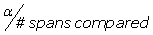UPT was appropriately sized but was computationally burdensome and, with current computing power, its application may not be reasonable in model building applications .

The type I error rates of ACST and CPT when applied with reduced significance cutoffs and the powers and sensitivities of ACST, CPT, FSPT, FMSPT and UPT have yet to be compared. In this study, we used simulated data to identify which hypothesis testing method, ACST, CPT, FSPT, FMSPT, or UPT, has the greatest power across a range of effect sizes under a simple alternative hypothesis. We examined the power curves of five global hypothesis testing methods. We estimated and compared the sensitivity and false positive rates of point-wise tests for the four permutation methods.

#### Methods

Simulated data

Data were simulated under a simple case-control setting. The study region was a circular subset of the Euclidean plane with a radius of one unit and a circular cluster located at its center. (Figure 1) This is a simplified version of a pattern that may be observed if subjects living within some radius of an exposure source, such as a lead smelter , are at constant increased or decreased risk when compared to subjects living further from the source.

Figure 1: Diagram of study region. Figure 1 is a diagram of the simulated study region. The circular cluster of increased or decreased risk is displayed in red. Observations falling in the blue area are considered “unexposed” and have a 20% probability of disease.

Two scenarios were considered where the cluster covered 15% (Scenario 1) or 5% (Scenario 2) of the study region. In both scenarios the probability of disease outside the cluster was held constant at 20%. Geographic locations were generated from a bivariate uniform distribution of longitude and latitude. For Scenario 1, odds ratios comparing subjects within to outside of the cluster were specified as 0.5, 1.0, 1.5, 2.0, 2.5 and 3.0. For the Scenario 2, odds ratios were 0.25, 1.0, 2.0, 3.0, 4.0 and 5.0. We chose these odds ratios to provide similar ranges of theoretical power estimates for Pearson chi-square tests applied to these scenarios while also reflecting odds ratios that may be observed in epidemiologic applications (Table 2, Table 3).

Hypothesis Testing Method Abbreviation Description Significance Cutoff
Approximate Chi-Square Test ACST Compare the model deviance statistic to an approximate chi-square distribution. 0.0125
Conditional Permutation Test CPT Select optimal span size for observed data by minimizing AIC statistic across range of spans. Compare difference in deviance statistic to conditional permutation distribution obtained by holding span size constant. 0.025
Fixed Span Permutation Test FSPT Select span size a priori. Compare difference in deviance statistic to conditional permutation distribution obtained by holding span size constant. 0.05
Fixed Multiple Span Permutation Test FMSPT Select 3-5 span sizes a priori. For each span size, compare the difference in deviance statistic to corresponding conditional permutation distribution obtained by holding the span size constant. Reject the null hypothesis if at least one p-value falls below the significance cutoff.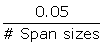Unconditional Permutation Test UPT Select optimal span size for observed data as in CPT. Compare difference in deviance statistic to unconditional permutation distribution obtained by selecting optimal span size for each permuted dataset. 0.05

Table 1: Description of Hypothesis Testing Methods and Significance Cutoffs.

 Odds Ratios 0.5 1.0 1.5 2.0 2.5 3.0 Significance Cutoff Power (95% CI) Type I Error (95% CI) Power (95% CI) Power (95% CI) Power (95% CI) Power (95% CI) Pearson Chi-Square Test Theoretical Power 0.05 0.731 (0.704,0.758) 0.050 (0.036,0.064) 0.521 (0.490,0.552) 0.953 (0.940,0.966) 0.999 (0.997,>0.999) >0.999 (0.997,>0.999) Observed Power 0.05 0.765 (0.739,0.791) 0.036 (0.024,0.048) 0.513 (0.482,0.544) 0.928 (0.912,0.944) 0.996 (0.992,>0.999) >0.999 (0.997,>0.999) ACST 0.0125 0.252 (0.225,0.279) 0.061 (0.046,0.076) 0.161 (0.138,0.184) 0.459 (0.428,0.490) 0.763 (0.737,0.789) 0.918 (0.901,0.935) CPT 0.025 0.239 (0.213,0.265) 0.047 (0.034,0.060) 0.149 (0.127,0.171) 0.447 (0.416,0.478) 0.764 (0.738,0.790) 0.923 (0.906,0.940) FSPT Span = 0.1 0.05 0.163 (0.140,0.186) 0.046 (0.033,0.059) 0.113 (0.093,0.133) 0.309 (0.280,0.338) 0.617 (0.587,0.647) 0.837 (0.814,0.860) Span = 0.3 0.05 0.234 (0.208,0.260) 0.043 (0.030,0.056) 0.150 (0.128,0.172) 0.415 (0.384,0.446) 0.755 (0.728,0.782) 0.917 (0.900,0.934) Span = 0.5 0.05 0.226 (0.200,0.252) 0.045 (0.032,0.058) 0.157 (0.134,0.180) 0.450 (0.419,0.481) 0.785 (0.76,0.810) 0.935 (0.920,0.950) Span = 0.7 0.05 0.223 (0.197,0.249) 0.042 (0.030,0.054) 0.153 (0.131,0.175) 0.453 (0.422,0.484) 0.787 (0.762,0.812) 0.923 (0.906,0.940) Span = 0.9 0.05 0.208 (0.183,0.233) 0.047 (0.034,0.060) 0.140 (0.118,0.162) 0.442 (0.411,0.473) 0.773 (0.747,0.799) 0.914 (0.897,0.931) FMSPT 0.1, 0.3, 0.5, 0.7, 0.9 0.01 0.152 (0.130,0.174) 0.020 (0.011,0.029) 0.094 (0.076,0.112) 0.330 (0.301,0.359) 0.673 (0.644,0.702) 0.880 (0.860,0.900) 0.1, 0.5, 0.9 0.0167 0.166 (0.143,0.189) 0.027 (0.017,0.037) 0.111 (0.092,0.130) 0.378 (0.348,0.408) 0.697 (0.669,0.725) 0.89 (0.871,0.909) UPT 0.05 0.088 (0.070,0.106) 0.045 (0.032,0.058) 0.094 (0.076,0.112) 0.263 (0.236,0.290) 0.545 (0.514,0.576) 0.789 (0.764,0.814)

Table 2: Scenario 1 Observed Power Estimates

 Odds Ratios 0.25 1.0 2.0 3.0 4.0 5.0 Significance Cutoff Power (95% CI) Type I Error (95% CI) Power (95% CI) Power (95% CI) Power (95% CI) Power (95% CI) Pearson Chi-Square Test Theoretical Power 0.05 0.693 (0.664,0.722) 0.050 (0.036,0.064) 0.622 (0.592,0.652) 0.971 (0.961,0.981) 0.999 (0.997,>0.999) >0.999 (0.997,>0.999) Observed Power 0.05 0.799 (0.774,0.824) 0.056 (0.042,0.07) 0.616 (0.586,0.646) 0.934 (0.919,0.949) 0.992 (0.986,0.998) >0.999 (0.997,>0.999) ACST 0.0125 0.175 (0.151,0.199) 0.059 (0.044,0.074) 0.145 (0.123,0.167) 0.345 (0.316,0.374) 0.526 (0.495,0.557) 0.722 (0.694,0.750) CPT 0.025 0.136 (0.115,0.157) 0.052 (0.038,0.066) 0.120 (0.100,0.14) 0.306 (0.277,0.335) 0.479 (0.448,0.510) 0.688 (0.659,0.717) FSPT Span = 0.1 0.05 0.168 (0.145,0.191) 0.044 (0.031,0.057) 0.177 (0.153,0.201) 0.287 (0.259,0.315) 0.471 (0.4400.502) 0.672 (0.643,0.701) Span = 0.3 0.05 0.145 (0.123,0.167) 0.047 (0.034,0.060) 0.125 (0.105,0.145) 0.321 (0.292,0.350) 0.504 (0.473,0.535) 0.711 (0.683,0.739) Span = 0.5 0.05 0.104 (0.085,0.123) 0.052 (0.038,0.066) 0.106 (0.087,0.125) 0.247 (0.220,0.274) 0.396 (0.366,0.426) 0.593 (0.563,0.623) Span = 0.7 0.05 0.103 (0.084,0.122) 0.053 (0.039,0.067) 0.101 (0.082,0.12) 0.222 (0.196,0.248) 0.363 (0.333,0.393) 0.517 (0.486,0.548) Span = 0.9 0.05 0.095 (0.077,0.113) 0.058 (0.044,0.072) 0.098 (0.080,0.116) 0.210 (0.185,0.235) 0.354 (0.324,0.384) 0.521 (0.490,0.552) FMSPT 0.1, 0.3, 0.5, 0.7, 0.9 0.01 0.081 (0.064,0.098) 0.026 (0.016,0.036) 0.073 (0.057,0.089) 0.219 (0.193,0.245) 0.382 (0.352,0.412) 0.587 (0.556,0.618) 0.1, 0.5, 0.9 0.0167 0.122 (0.102,0.142) 0.04 (0.028,0.052) 0.100 (0.081,0.119) 0.268 (0.241,0.295) 0.443 (0.412,0.474) 0.648 (0.618,0.678) UPT 0.05 0.161 (0.138,0.184) 0.041 (0.029,0.053) 0.125 (0.105,0.145) 0.275 (0.247,0.303) 0.463 (0.432,0.494) 0.650 (0.620,0.680)

Table 3: Scenario 2 Observed Power Estimates.

Odds ratios of 0.25 and 0.5 indicate locations of decreased risk while odds ratios greater than 1.0 indicate areas of increased risk. For each of these odds ratios, 1,000 datasets were simulated, each containing 1,000 observations. We selected the dichotomous outcome and sample size to reflect previous studies in spatial epidemiology that used GAMs as a primary statistical method [22-24]. The nominal type I error rate for each test was 0.05. All simulations and analyses were performed using the statistical software R v2.8.0. . Syntax used to generate synthetic data is available on the Boston University Superfund Basic Research Program website (http://www.busrp.org/).

Theoretical power

The data could be analyzed using a Pearson chi-square test though, in practice, investigators would not be aware that the association was not more complex. To evaluate the performance of the hypothesis testing methods, we computed the theoretical power for a Pearson chi-square test: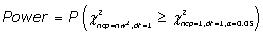,where ncp is the noncentrality parameter equal to the sample size multiplied by the effect size, w, squared with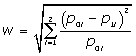Here, P01and P02 are the joint probabilities of controls living inside and outside the cluster, while P11 and P12 are the joint probabilities of cases living inside and outside the cluster . The theoretical powers ranged from 0.050 to >0.999. (Table 2) Pearson chi-square tests were performed on the simulated datasets for each parameter combination. Simulated power for this test was defined as the proportion of datasets where we rejected the null hypothesis with a significance level of 0.05.

Hypothesis testing methods

GAMs were applied to simulated datasets using a bivariate LOESS smoothing term to adjust for geographic location  using of the gam package  in R v2.8.0. . When necessary we applied GAMs across a range of span sizes between 0.05 and 0.95 and selected the span that minimized the model AIC . As cluster size differed in the two scenarios, we expected the distributions of selected spans to differ as well.

For ACST, it was shown through simulations that the distribution of difference in deviance statistics of models applied with and without smoothing terms can be loosely approximated by a chi-square distribution . In this application, when applying ACST, for each dataset, the span size was selected and the approximate statistic and p-value were recorded. The test was found to have an inflated type I error rate when the significance cut-off was 0.05 with an observed rejection rate of 0.151 (95% CI: 0.137-0.165) under the null hypothesis . Intuitively, one might divide α by 3 as the observed type I error was approximately 3 times greater than the desired; however this adjustment provided an observed type I error rate of 0.073 (95% CI: 0.059-0.087) and did not correct the test size. Dividing α by 4 may seem conservative; however simulations showed that this adjustment provided a test of the appropriate size (0.061; 95% CI: 0.047-0.075).

For CPT, the span size was selected for each dataset and the difference in deviances between models including and excluding the bivariate LOESS smoothing term was computed. Through permutation of geographic locations, 999 permuted datasets were created and GAMs were applied. For each permuted dataset the difference in deviance statistic was recorded, conditioned on the selected span for the observed data. The statistics were ranked and the global null hypothesis of no association between the outcome and smoothed term was rejected if the observed difference in deviance fell in the upper tail of the conditional permutation distribution . The CPT was found to have an inflated type I error rate when applied with a significance cut-off of 0.05 and a rejection rate of 0.090 (95% CI: 0.076-0.104) . We adjusted the nominal cutoff to the upper 2.5% to obtain an observed type I error rate of the correct level.

The FSPT was applied across five span sizes: 0.1, 0.3, 0.5, 0.7 and 0.9, selected to display power estimates across a range of possible span sizes. GAMs with each predetermined span size were applied to observed and permuted datasets. The power for each of the five spans was obtained by rejecting the null hypothesis when the observed statistic fell in the upper 5% of the corresponding permutation distribution . We also evaluated the power of a hypothesis test considering the data at multiple span sizes, the Fixed Multiple Span Permutation Test (FMSPT). For combinations of three or five spans across the range of possible span sizes we rejected the null hypothesis if any of the observed statistics fell in the upper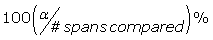of the corresponding permutation distribution. The adjustment was suggested in a previous paper to obtain tests of the appropriate size .

We selected the span size by minimizing the model AIC for observed and permuted datasets to perform the UPT. We rejected the null hypothesis if the observed difference in deviance statistic fell in the upper 5% of the permutation distribution .

Point-wise hypothesis tests

For each permutation method, point-wise hypothesis tests were performed by recording the predicted logodds from observed and permuted datasets at each point on a fine regular grid (1955 points per unit circle) overlaying the study region. Permutation distributions of point-wise predicted logodds were produced for each point. Hotspots, areas of increased risk, were identified as locations having predicted logodds from the observed data that fell in the upper 2.5% of the corresponding permutation distribution of predicted logodds. Coldspots, areas of decreased risk, were identified as locations with predicted logodds that fell in the lower 2.5% of the distribution . It is unclear whether the conditional or unconditional nature of the permutation tests will affect the results. A point-wise testing method is not available for the ACST.

Sensitivity and false positive rate were defined in a similar manner to Ozonoff et al.  when evaluating local hypothesis tests, sensitivity was defined as the conditional proportion of the true cluster correctly identified as increased or decreased risk, given the global null hypothesis was rejected [28,29].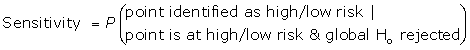False positive rate, the complement of specificity, was defined as the proportion of the study region falsely detected by the methods as high or low risk when the global hypothesis was rejected.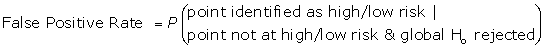Note that the false positive rate can be computed under the null hypothesis while sensitivity depends on the choice of a specific alternative.

#### Results

The observed power estimates for the Pearson chi-square test were similar to the theoretical power for both Scenarios 1 and 2. (Table 2, Table 3) ACST had observed type I error rates of 0.061 (95% CI: 0.046-0.076) and 0.059 (95% CI: 0.044-0.074) when applied under the null hypothesis. The nominal level of 0.05 fell within a 95% confidence interval of the ACST estimates as well as for the type I error estimates of CPT (Scenario 1: 0.047; 0.034-0.060; Scenario 2: 0.052; 0.038-0.066). The FSPT, FMSPT and UPT had observed type I error rates at or below the nominal level (Table 2, Table 3).

The power estimates for ACST under alternative hypotheses were smaller than the theoretical power, ranging between 0.161 and 0.918 for Scenario 1 and 0.145 and 0.722 for Scenario 2. The CPT had power of similar magnitude to the ACST with estimates ranging from 0.149 to 0.923 and 0.120 to 0.688 for Scenarios 1 and 2, respectively. (Table 2, Table 3) Compared to their application with an unadjusted significance cutoff of 0.05, the ACST and CPT had reduced power across effect sizes. The shapes of the respective power curves were similar, regardless of the significance cutoffs for each method (Figure 2, Figure 3).

Figure 2: Power curves of approximate chi-square test with nominal and adjusted significance cutoffs in Scenario 1. Figure 2 displays observed power curves and 95% confidence bands when ACST was applied with nominal and adjusted significance cutoffs in Scenario 1.

Figure 3: Power curves of conditional permutation test with nominal and adjusted significance cutoffs in Scenario 1. Figure 3 displays observed power curves and 95% confidence bands when CPT was applied with nominal and adjusted significance cutoffs in Scenario 1.

For Scenario 1, the distribution of spans selected for observed data through the minimization of the AIC statistic were left skewed with a single mode near spans of 0.9 for odds ratios of less than 2.0. For odds ratios of at least 2.0 the distributions were bimodal with modes near 0.3 and 0.6. (Figure 4) For Scenario 2, odds ratios less than 3.0 had left skewed distributions of optimal spans while larger odds ratios corresponded to an increased density for span sizes near 0.2 (Figure 5).

Figure 4: Distributions of selected span size across odds ratios in Scenario 1. Figure 4 displays the distribution of span sizes observed to minimize the AIC statistic when GAMs were applied in Scenario 1. This is also the distribution of span sizes selected for the application of ACST, CPT and UPT.

Figure 5: Distributions of selected span size across odds ratios in Scenario 2. Figure 5 displays the distribution of span sizes observed to minimize the AIC statistic when GAMs were applied in Scenario 2. This is also the distribution of span sizes selected for the application of ACST, CPT and UPT.

In Scenario 1, with a cluster covering 15% of the study region, the FSPT showed unbiased type I error rates across all span sizes and had power estimates ranging from around 0.150 to at least 0.900 for spans greater than 0.1. Estimates for a span of 0.1 were slightly lower. The highest power was observed for spans of 0.5 and 0.7. (Table 2) For a cluster covering 5% of the study region (Scenario 2), the greatest power was observed for a span of 0.3 followed by spans of 0.1 and 0.5. (Table 3) Evaluated at multiple spans, the FMSPT performed well with power estimates slightly smaller than those of the FSPT. The slight power reduction was likely due to the conservative significance cutoff: α divided by the number of spans compared. The observed type I error rates were conservative for both scenarios. The power estimates ranged from less than 0.100 to approximately 0.900 for Scenario 1 and from less than 0.100 to 0.650 for Scenario 2 (Table 2, Table 3).

UPT had reduced power when compared to the other methods in Scenario 1. Power estimates ranged from around 0.100 to near 0.800, smaller than estimates for FSPT. In Scenario 2, UPT performed better than FSPT with spans of 0.5, 0.7 and 0.9 while it had comparable power estimates to the FSPT with a span of 0.1. The power estimates ranged from 0.125 to 0.650 (Table 2, Table 3).

Comparing computing times, using a personal computer with 504MB RAM, for a single analysis on a dataset including 1,000 observations, CPT, FSPT and FMSPT were completed in less than 15 minutes. For the same analysis, UPT was completed in 5.5 hours.

Examining power curves for Scenarios 1 and 2, ACST and the permutation tests had fairly similar power estimates across the range of effect sizes though they were outperformed by the theoretical and observed Pearson chi-square tests. In Scenario 1, the greatest power was observed for the ACST and CPT however there was little difference between these tests and the power of the fixed spans of 0.5 and 0.7. (Figure 6) In Scenario 2, maximum power was obtained by the ACST, closely followed by the FSPT for a span of 0.3 and similar estimates were observed for a span of 0.1, the CPT and the UPT (Figure 7).

Figure 6: Power curves of all hypothesis testing methods in Scenario 1. Figure 6 displays the power curves of the theoretical power, Pearson’s chisquare test, ACST, CPT, FSPT, FMSPT and UPT when applied to Scenario 1.

Figure 7: Power curves of all hypothesis testing methods in Scenario 2. Figure 7 displays the power curves of the theoretical power, Pearson’s chisquare test, ACST, CPT, FSPT, FMSPT and UPT when applied to Scenario 2.

Sensitivity and false positive rates for the permutation based methods are presented in Tables 4 and 5. Scenarios 1 and 2 shared false positive rates of approximately 5% under the null hypothesis, a trend of increasing false positive rates with increasing effect size and higher false positive rates for the UPT with respect to several other methods (Table 4, Table 5).

 Odds Ratios 0.5 1.0* 1.5 2.0 2.5 3.0 Sensitivity False Positive False Positive Sensitivity False Positive Sensitivity False Positive Sensitivity False Positive Sensitivity False Positive Mean (Median;Std) Mean (Median;Std) Mean (Median;Std) Mean (Median;Std) Mean (Median;Std) Mean (Median;Std) Mean (Median;Std) Mean (Median;Std) Mean (Median;Std) Mean (Median;Std) Mean (Median;Std) CPT 0.88 (>0.99;0.27) 0.14 (0.11;0.13) 0.06 (0;0.09) 0.65 (0.91;0.4) 0.11 (0.09;0.12) 0.93 (>0.99;0.21) 0.21 (0.21;0.13) 0.98 (>0.99;0.1) 0.28 (0.28;0.11) 0.99 (>0.99;0.08) 0.31 (0.3;0.11) FSPT Span = 0.1 0.55 (0.6;0.35) 0.08 (0.08;0.05) 0.05 (0.05;0.03) 0.38 (0.26;0.36) 0.07 (0.06;0.04) 0.64 (0.74;0.34) 0.10 (0.10;0.04) 0.78 (0.91;0.27) 0.14 (0.14;0.04) 0.89 (>0.99;0.21) 0.17 (0.17;0.04) Span = 0.3 0.91 (>0.99;0.2) 0.11 (0.1;0.09) 0.05 (0.04;0.06) 0.66 (0.79;0.37) 0.08 (0.07;0.07) 0.96 (>0.99;0.12) 0.14 (0.14;0.08) 0.99 (>0.99;0.06) 0.20 (0.20;0.07) >0.99 (>0.99;0.02) 0.24 (0.24;0.06) Span = 0.5 0.89 (>0.99;0.27) 0.14 (0.12;0.12) 0.05 (0.01;0.07) 0.75 (0.98;0.34) 0.10 (0.09;0.10) 0.97 (>0.99;0.13) 0.20 (0.21;0.12) 0.99 (>0.99;0.06) 0.29 (0.30;0.09) >0.99 (>0.99;0.01) 0.35 (0.36;0.08) Span = 0.7 0.86 (>0.99;0.31) 0.15 (0.13;0.14) 0.05 (0;0.08) 0.77 (>0.99;0.36) 0.12 (0.09;0.12) 0.96 (>0.99;0.14) 0.24 (0.26;0.14) 0.99 (>0.99;0.07) 0.35 (0.37;0.1) >0.99 (>0.99;0.01) 0.41 (0.42;0.08) Span = 0.9 0.84 (>0.99;0.33) 0.13 (0.1;0.13) 0.05 (0;0.09) 0.78 (>0.99;0.36) 0.11 (0.07;0.12) 0.95 (>0.99;0.2) 0.22 (0.24;0.14) 0.99 (>0.99;0.07) 0.33 (0.35;0.11) >0.99 (>0.99;0.01) 0.39 (0.39;0.08) UPT 0.95 (>0.99;0.15) 0.13 (0.04;0.16) 0.05 (0;0.10) 0.76 (0.95;0.33) 0.10 (0.01;0.13) 0.97 (>0.99;0.1) 0.22 (0.24;0.16) >0.99 (>0.99;0.03) 0.33 (0.35;0.12) >0.99 (>0.99;0.03) 0.38 (0.39;0.09)

Table 4: Scenario 1 Sensitivity and False Positive Rates of Permutation Test Methods under Alternative Hypotheses.

 Odds Ratios 0.25 1.0* 2.0 3.0 4.0 5.0 Sensitivity False Positive False Positive Sensitivity False Positive Sensitivity False Positive Sensitivity False Positive Sensitivity False Positive Mean (Median;Std) Mean (Median;Std) Mean (Median;Std) Mean (Median;Std) Mean (Median;Std) Mean (Median;Std) Mean (Median;Std) Mean (Median;Std) Mean (Median;Std) Mean (Median;Std) Mean (Median;Std) CPT 0.73 (0.95;0.39) 0.09 (0.05;0.11) 0.07 (0;0.10) 0.64 (0.79;0.38) 0.08 (0.04;0.11) 0.86 (>0.99;0.27) 0.13 (0.11;0.11) 0.97 (>0.99;0.1) 0.15 (0.13;0.11) 0.99 (>0.99;0.08) 0.16 (0.14;0.10) FSPT Span = 0.1 0.71 (0.84;0.33) 0.06 (0.06;0.04) 0.05 (0.05;0.03) 0.58 (0.67;0.32) 0.06 (0.05;0.03) 0.87 (0.95;0.2) 0.07 (0.07;0.04) 0.96 (>0.99;0.09) 0.08 (0.08;0.03) 0.98 (>0.99;0.06) 0.09 (0.09;0.03) Span = 0.3 0.72 (0.88;0.36) 0.07 (0.06;0.07) 0.06 (0.04;0.06) 0.61 (0.79;0.39) 0.07 (0.05;0.06) 0.88 (>0.99;0.25) 0.10 (0.09;0.07) 0.97 (>0.99;0.11) 0.12 (0.11;0.06) 0.99 (>0.99;0.07) 0.14 (0.14;0.06) Span = 0.5 0.70 (0.93;0.38) 0.08 (0.05;0.09) 0.06 (0.02;0.08) 0.65 (0.86;0.40) 0.08 (0.04;0.09) 0.80 (>0.99;0.33) 0.13 (0.12;0.11) 0.94 (>0.99;0.17) 0.17 (0.17;0.11) 0.96 (>0.99;0.16) 0.21 (0.22;0.11) Span = 0.7 0.64 (0.98;0.43) 0.09 (0.03;0.11) 0.06 (0;0.09) 0.67 (0.95;0.41) 0.09 (0.03;0.11) 0.83 (>0.99;0.32) 0.16 (0.15;0.13) 0.92 (>0.99;0.2) 0.22 (0.23;0.13) 0.97 (>0.99;0.11) 0.27 (0.29;0.13) Span = 0.9 0.68 (>0.99;0.43) 0.08 (0;0.11) 0.06 (0;0.10) 0.61 (0.79;0.43) 0.08 (0;0.11) 0.83 (>0.99;0.32) 0.14 (0.12;0.13) 0.90 (>0.99;0.24) 0.19 (0.20;0.13) 0.96 (>0.99;0.15) 0.24 (0.26;0.13) UPT 0.86 (>0.99;0.26) 0.10 (0;0.15) 0.05 (0;0.10) 0.79 (0.91;0.29) 0.08 (0;0.13) 0.97 (>0.99;0.12) 0.15 (0.12;0.15) 0.99 (>0.99;0.03) 0.21 (0.23;0.15) >0.99 (>0.99;0.04) 0.26 (0.28;0.14)

Table 5: Scenario 2 Sensitivity and False Positive Rates of Permutation Test Methods under Alternative Hypotheses.

For Scenario 1, the highest sensitivity rates were observed for FSPT with spans of 0.5 and 0.7 and the UPT. The CPT also performed well detecting, on average, between 65 and 99% of the true cluster. The lowest sensitivity was observed for the FSPT with a span of 0.1. False positive rates were between 5 and 40% for the permutation tests. Overall, the FSPT with a span of 0.1 had the lowest false positive rates while the span of 0.7 had the highest rates across effect sizes (Table 4).

In Scenario 2, the highest sensitivity was observed for the UPT, followed closely by the CPT (97%) and the FSPT with spans of 0.1 and 0.3. Across all effect sizes, the sensitivity estimates were similar for all tests. False positive rates increased with increasing effect sizes. Consistently high false positive rates were observed for the UPT followed by the FSPT with spans of 0.7 and 0.9. Lowest false positive rates were observed for the span of 0.1 followed by the span of 0.3 with the CPT also showing low values (Table 5).

#### Discussion

In this paper the type I error rates and powers of five hypothesis testing methods were compared to theoretical and observed type I error and power of Pearson chi-square tests in two simple scenarios. In Scenario 1, a circular cluster covered 15% of the circular study region area, representing a large cluster close to the size of Worcester County in Massachusetts, while in Scenario 2, the circular cluster covered 5% of the area representing a small cluster the size of Barnstable County in Massachusetts . In previous research we performed a power comparison of CPT, FMSPT and the spatial scan statistic. The relative pattern of power estimates for CPT and FMSPT was preserved through changes in region shape and variation in disease risk . Similar results would be expected for ACST, FSPT and UPT. The pattern of disease risk for this paper was selected to present a simple alternative hypothesis where the tests were expected to have high power.

The Pearson chi-square test, a simple but theoretically appropriate test, outperformed the ACST and all permutation tests in its observed and theoretical power. These results are not surprising considering the simplicity of the Pearson test and the use of added information about a dichotomous exposure pattern. It is of note that in Scenario 1, with a large cluster, the ACST, CPT and some FSPTs had power estimates nearing those of the Pearson chi-square test.

The ACST and CPT were shown to have inflated type I error rates in previous research . In this paper, we applied empirically based significance cutoff adjustments to provide appropriately sized tests. While there is not sufficient evidence to guarantee these adjustments will hold in future studies, initial evidence based on unpublished research indicates the adjustments are robust to region shape and variations in population densities.

The ACST and CPT, when applied with reduced significance cutoffs, had high power estimates when compared to other methods in both scenarios and across effect sizes. For Scenario 1, the highest power was observed for FSPT with mid-ranged span sizes of 0.5 and 0.7 while for Scenario 2, the most often selected span size was smaller, corresponding to high power estimates for FSPT with spans of 0.1 and 0.3. Comparing power estimates across span sizes, the choice of span for FSPT does not affect the type I error rate; however the span was observed to influence the power of the hypothesis test. FSPT using spans with high densities in Figures 4 and 5 correspond to higher power estimates than for lower density spans. To maximize power, researchers would select a span expected to minimize the AIC statistic for the data at hand. In practice, however, the distribution of selected spans is unknown making the a priori selection of a span to minimize the AIC difficult and likely reducing the power of FSPT.

FMSPT in Scenario 1 showed power estimates smaller than most FSPTs, exceeding only the estimate for a span of 0.1; while in Scenario 2, the power estimates for the FMSPT were greater than all but the fixed spans of 0.1 and 0.3. In both scenarios, the evaluation of the FMSPT produced adequate power in relation to the estimates for a single fixed span, despite its conservative type I error rate.

It is of interest that the UPT was outperformed by the FSPT for all span sizes in Scenario 1 while it had higher power than spans of 0.5 or greater in Scenario 2. When applying the UPT, the critical value for the significance cutoff is determined as the 95th percentile of the ranked deviance statistics, obtained from permuted datasets. In general, the difference in deviance statistics obtained from GAMs with large spans will be smaller than those observed with small spans. Applying the UPT, for each permuted dataset, the span size is selected through the minimization of the AIC statistic. Under the null hypothesis the most appropriate span size is the largest available, i.e. the closest value to 1. By chance, some permuted datasets have characteristics causing a smaller span size to be selected and, as a result, the unconditional permutation distribution has larger variation than would be observed for a conditional permutation distribution. The rank of the observed statistic in an unconditional permutation distribution is often smaller, i.e. farther to the left, than the ranked statistic in a fixed span permutation distribution. As a result, the null hypothesis is rejected less frequently, corresponding to reduced power for the UPT for a fixed span test.

Though small span sizes may be observed in practice, in the extreme case examined in Scenario 2 with an odds ratio of 5.0, the UPT did not perform much better than the FSPT when applied with fixed large span sizes. Additionally, it was outperformed by the CPT and the FMPST had power estimates nearing those of the UPT.

In Scenario 1, with effect sizes of 2.0 or greater, the UPT and FSPT with span sizes of 0.3 or greater had sensitivity rates of at least 80%. In Scenario 2, with odds ratios of at least 3.0, all tests had sensitivity estimates of at least 80%. Sensitivity of this magnitude or greater indicates that at least 80% of the area that was truly at risk was detected by these methods, a reasonable requirement of a statistical test in practice. Increased sensitivity increases researchers’ abilities to detect areas that are at risk and subsequently provide services to those neighborhoods.

In contrast, researchers aim to reduce false positive rates as increased false detection in spatial epidemiologic studies may waste resources as public health officials target unaffected areas with unnecessary procedures to reduce residential risk. Depending on the severity of the disease of interest, risk of exposure to residents and cost of resources sent to detected areas, different false positive rates may be acceptable to researchers. In Scenario 1, the highest false positive rate was observed for a fixed span of 0.7 with 41% false positives for an odds ratio of 3.0. Depending on the costs, this rate may be considered high and the CPT may be preferred as its false positive rate was smaller at 31%. For Scenario 2, the highest false positive rates were observed for fixed spans of 0.7, 0.9 and for UPT. The CPT outperformed many of the methods with smaller rates than the UPT and the FSPT with spans of at least 0.5. In general, with increased sensitivity comes an increased false positive rate as the two measures are highly related. Researchers must balance the benefits of the sensitivity of a test with the false positive rate in order to select a desirable test that can detect areas of increased risk without extreme false positive rates.

In this study, we considered two simple scenarios with different most appropriate span sizes, one large and one small, expected to minimize the AIC. We included an array of effect sizes and obtained a wide range of observed power estimates. We computed theoretical power using a Pearson chi-square test for comparison to the testing methods performed with GAMs. Though we included only one cluster pattern, other research has indicated that different variations in disease risk are not expected to change the relative patterns of power and sensitivity . Benchmark data is provided on the Boston University Superfund Basic Research Group website (http:// www.busrp.com) to allow comparison of GAMs with other methods; however an extensive power comparison in complex scenarios, such as areas with sparse data, irregular boundaries and multiple clusters, is left for future research. While we considered span selection by minimizing the AIC statistic, there are many other methods that can be used. We expect similar patterns of relative power to be observed for any data driven span selection procedures relying on functions of model deviance. The confirmation of this is left to future research.

We proposed type I error rate adjustments for the ACST and CPT based on empirical evidence and a nominal level of 0.05. While the adjustments produced tests of an appropriate size in this application, for other nominal levels simulation studies must be performed to determine appropriate adjustments. Though not rigorously proven, we present an explanation for the relative positioning of the hypothesis testing methods in a hierarchy of power, sensitivity and false positive estimates based on the results of this study. The evaluation of sensitivity and false positive rates for FMSPT is left for future research.

#### Conclusion

The choice of hypothesis testing method may depend on the motivation of the analysis. For exploratory or model building investigations, ACST, CPT, FSPT and FMSPT are appropriate. The statistic and p-value for ACST are produced by standard software and, when applied with a reduced significance cutoff, the test had high power estimates; however ACST is disadvantaged in the limited information it provides. Permutation tests can detect overall variation in outcomes across the study region and can identify specific areas of increased or decreased risk while ACST can only evaluate overall departures from the null hypothesis of spatial randomness. CPT uses data driven span selection procedures, allowing investigators to gain information about the degree of spatial variation in the study region, to see how this may change in a model building scenario, as well as to perform hypothesis tests. When applied with a reduced significance cutoff it is appropriately sized and produces high power estimates. FSPT is appropriately sized; however it requires the selection of a single span and its power depends on the span size. FMSPT is a better alternative, allowing investigators to produce maps using multiple span sizes and to examine possible associations at a variety of smoothing levels. Though conservative in its type I error rate, FMSPT produced adequate power estimates. UPT, mathematically, is the most appropriate test and may be applied when investigators would like to avoid a priori span size selections while also maintaining the nominal type I error rate without applying significance cutoff adjustments. However, in light of its computational burden, UPT may not be appropriate for model building scenarios at this time as CPT, FSPT and FMSPT can provide more immediate results.

#### Acknowledgement

This research was supported by grant P42ES007381 from the National Institute of Environmental Health (NIEHS), NIH and grant 5T32AR055885-03 from the National Institute of Arthritis and Musculoskeletal and Skin Diseases (NIAMS), NIH. Its contents are solely the responsibility of the authors and do not necessarily represent the official views of NIEHS, NIAMS, or NIH.

#### References

Select your language of interest to view the total content in your interested language

### Article Usage

• Total views: 12284
• [From(publication date):
November-2010 - Aug 19, 2019]
• Breakdown by view type
• HTML page views : 8472
• PDF downloads : 3812

## Post your commentCan't read the image? click here to refresh
###### Peer Reviewed Journals

Make the best use of Scientific Research and information from our 700 + peer reviewed, Open Access Journals

International Conferences 2019-20

Meet Inspiring Speakers and Experts at our 3000+ Global Annual Meetings

Top